# 太强了，竟然可以根据指纹图像预测性别！

08/21 10:05• 了解数据集

• 重新构造数据集（以便使用keras 的Flow_from_directory函数）

• 定义一个简单的函数提取所需的特定标签

• 定义一个简单的函数读取图像、调整图像大小。

• 预处理训练和测试数据

• 从头开始构建简单的CNN模型

• 训练和测试模型

https://www.freecodecamp.org/news/an-intuitive-guide-to-convolutional-neural-networks-260c2de0a050/

•这篇文章假定您具有卷积神经网络（CNN）的知识。

•该代码是在kaggle内核中执行的。它提供免费的GPU和RAM，不足之处是空间有限，但您可以轻松删除不需要的变量。

https://www.kaggle.com/ruizgara/socofing

• 它有两个主目录-Real目录和Altered目录

• Real目录包含真实人类指纹（无任何变化）

• Altered目录包含经过综合更改的指纹图像，包括用于遮盖中央旋转和Z形切割的三种不同级别的更改。

• Altered目录进一步分为：Altered_EasyAltered_hardAltered_Medium目录

• Real图像的标签如下所示：“ 100__M_Left_thumb_finger.BMP”

• Altered图像的标签遵循以下格式：“ 100__M_Left_thumb_finger_CR.BMP”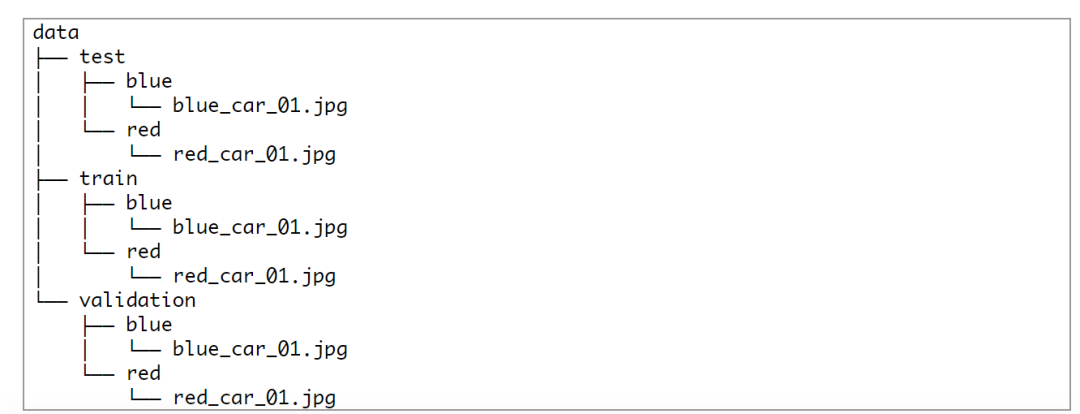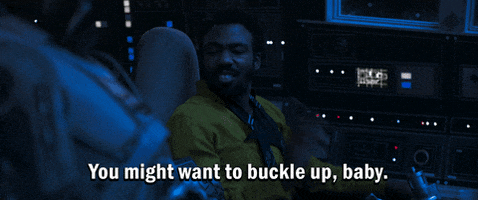“ 100__M_Left_thumb_finger_CR.BMP”

“ 100__M_Left_thumb_finger.BMP”

````#import necessary libraries``import numpy as np``import pandas as pd``import seaborn as sns``import tensorflow as tf``import os``import cv2``import matplotlib.pyplot as plt``#Function to extract labels for both real and altered images``def extract_label(img_path,train = True):``  filename, _ = os.path.splitext(os.path.basename(img_path))`
`  subject_id, etc = filename.split('__')``  #For Altered folder``  if train:``      gender, lr, finger, _, _ = etc.split('_')``  #For Real folder``  else:``      gender, lr, finger, _ = etc.split('_')``  ``  gender = 0 if gender == 'M' else 1``  lr = 0 if lr == 'Left' else 1`
`  if finger == 'thumb':``      finger = 0``  elif finger == 'index':``      finger = 1``  elif finger == 'middle':``      finger = 2``  elif finger == 'ring':``      finger = 3``  elif finger == 'little':``      finger = 4``  return np.array([gender], dtype=np.uint16)````

``img_size = 96``#Function to iterate through all the images``def loading_data(path,train):``    print("loading data from: ",path)``    data = []``    for img in os.listdir(path):``        try:``            img_array = cv2.imread(os.path.join(path, img), cv2.IMREAD_GRAYSCALE)``            img_resize = cv2.resize(img_array, (img_size, img_size))``            label = extract_label(os.path.join(path, img),train)``            data.append([label, img_resize ])``        except Exception as e:``            pass``    data``    return data``

• 首先设定预期的图像尺寸 img_size = 96。

• 迭代：对路径（path）中的所有图像进行操作——读取图像并将它们转换为灰度图像（即黑白），然后将这些图像的像素值数组返回到img_array

• img_resize包含已基于img_size调整大小的数组值。因此所有图像将具有相同的大小（96x96）。

• 调用extract_labels函数来获取标签，label中包含标签值。

• 所有标签和调整大小的图像数组添加到data列表 。

• 使用try, except块，传递异常

````Real_path = "../input/socofing/SOCOFing/Real"``Easy_path = "../input/socofing/SOCOFing/Altered/Altered-Easy"``Medium_path = "../input/socofing/SOCOFing/Altered/Altered-Medium"``Hard_path = "../input/socofing/SOCOFing/Altered/Altered-Hard"`

`Easy_data = loading_data(Easy_path, train = True)``Medium_data = loading_data(Medium_path, train = True)``Hard_data = loading_data(Hard_path, train = True)``test = loading_data(Real_path, train = False)`
`data = np.concatenate([Easy_data, Medium_data, Hard_data], axis=0)`
`del Easy_data, Medium_data, Hard_data````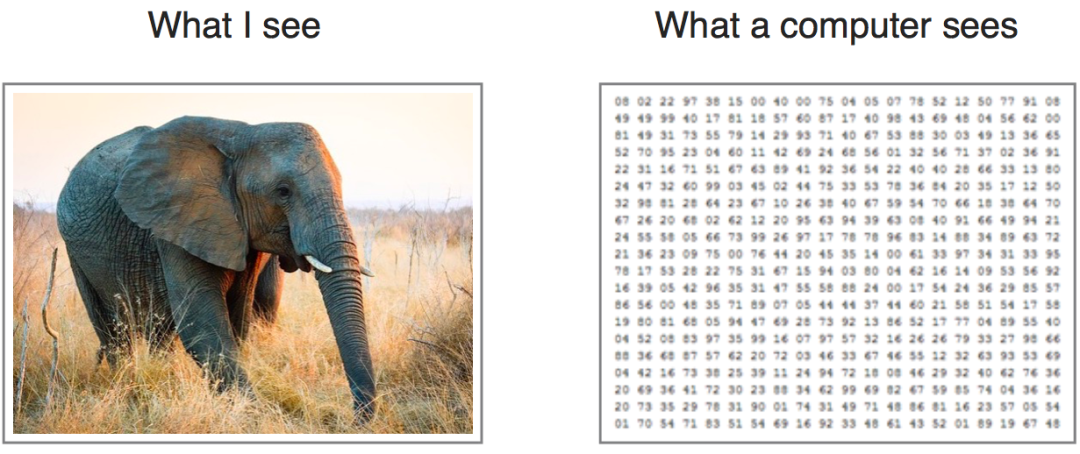（1）随机化训练数据data和测试数据test数组。并查看data的格式

``import random``random.shuffle(data)``random.shuffle(test)``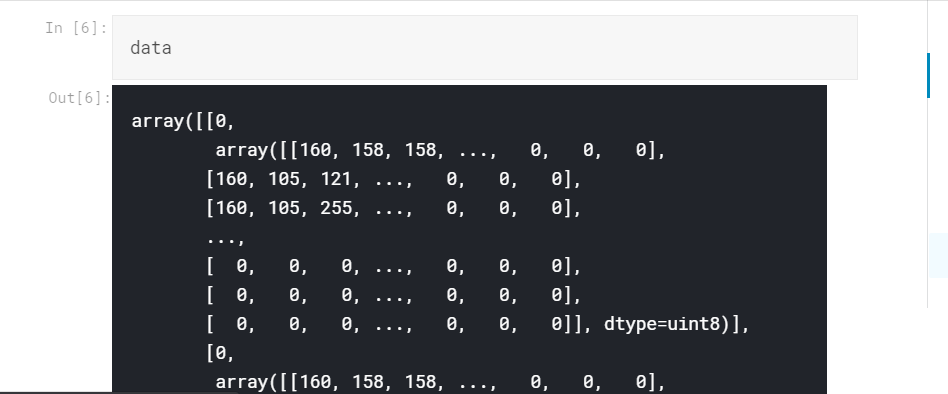（2）分离图像数组和标签

``img, labels = [], []``for label, feature in data:``    labels.append(label)``    img.append(feature)``train_data = np.array(img).reshape(-1, img_size, img_size, 1)``train_data = train_data / 255.0``from keras.utils.np_utils import to_categorical``train_labels = to_categorical(labels, num_classes = 2)``del data``

• 使用for循环，将图像数组和标签分成单独的列表

• img包含图像数组，labels包含标签值

• img和 labels是列表

• img中的值在重新调整之前再次转换为数组

• 图像的像素值的范围是0到255，通过除以255.0，像素值将按比例缩小到0到1，变为train_data。

• 标签也从列表转换为分类值，我们有两个类F和M类，分配给train_labels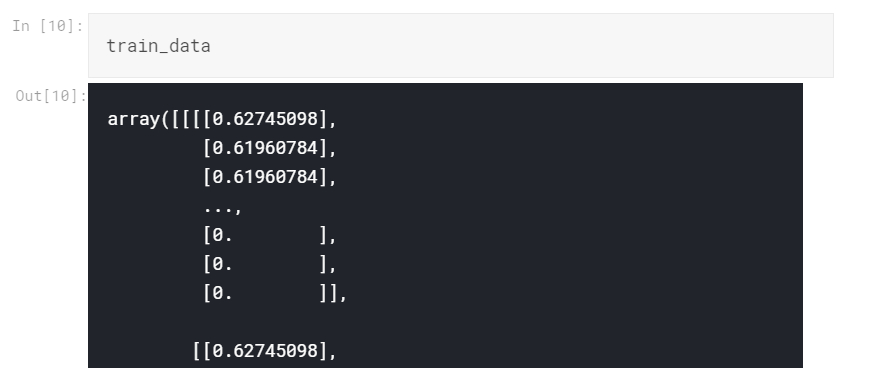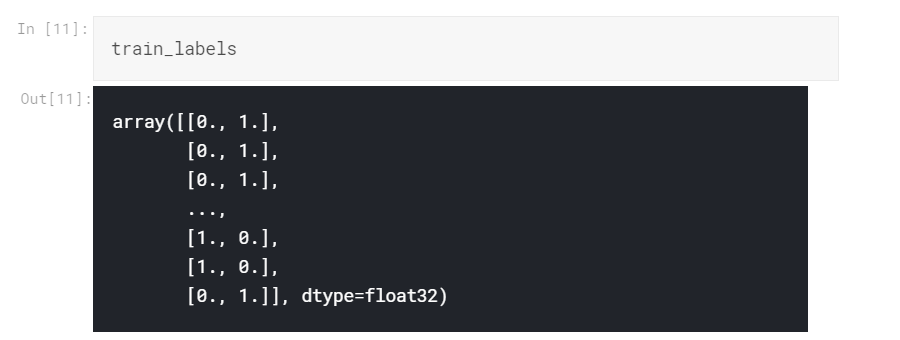（3）最后一步，使用训练、验证和测试数据集来训练模型。已经有了训练和测试数据，我们仍然需要验证数据（test），因此我们可以使用来自sklearn库的train_test_splitfrom或使用keras的validation_split设置验证数据。（1）构建模型网络结构

• 使用tensorflow来构建我们的模型

• 从头开始构建一个简单的CNN模型，在每层都有两个卷积层之后将通过relu激活函数添加一个max pooling层

• 之后添加一个flatten层、一个隐藏密集层，然后是一个输出层。

• input_shape = [96,96,1]（1是我们的灰度图像的结果）

• 类别数量2

````#Import necessary libraries``from tensorflow.keras import Sequential ``from tensorflow.keras.layers import Conv2D, MaxPooling2D, Dense, Flatten``from tensorflow.keras import layers``from tensorflow.keras import optimizers`
`model = Sequential([``                    Conv2D(32, 3, padding='same', activation='relu',kernel_initializer='he_uniform', input_shape = [96, 96, 1]),``                    MaxPooling2D(2),``                    Conv2D(32, 3, padding='same', kernel_initializer='he_uniform', activation='relu'),``                    MaxPooling2D(2),``                    Flatten(),``                    Dense(128, kernel_initializer='he_uniform',activation = 'relu'),``                    Dense(2, activation = 'softmax'),``                    ])````

•  每个卷积层（Conv2D）包含32个大小为3（即3 x 3）的过滤器，仅在第一层中设置输入形状

•  Max pooling（MaxPooling2D）的池化大小为2

•  只有一个具有128个神经单元的隐藏层（Dense），激活函数是relu

•  分类：将使用Dense大小为2（类编号）的输出层和softmax激活来结束网络。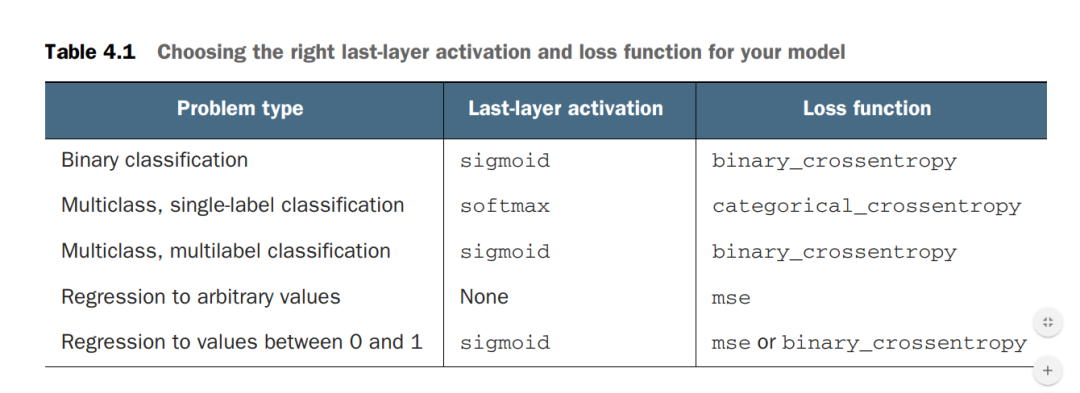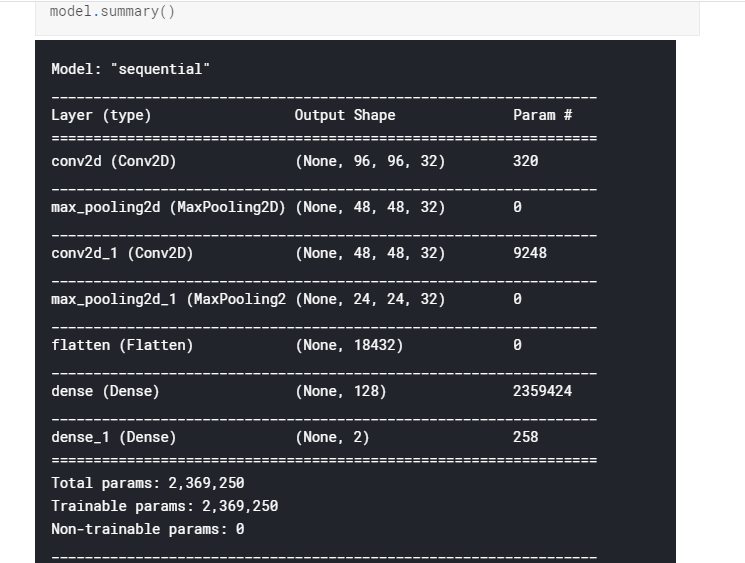``model.compile(optimizer = optimizers.Adam(1e-3), loss = 'categorical_crossentropy', metrics = ['accuracy'])``early_stopping_cb = tf.keras.callbacks.EarlyStopping(monitor='val_loss', patience=10)``

（2）编译模型：

（3）拟合模型

``history = model.fit(train_data, train_labels, batch_size = 128, epochs = 30, ``          validation_split = 0.2, callbacks = [early_stopping_cb], verbose = 1)``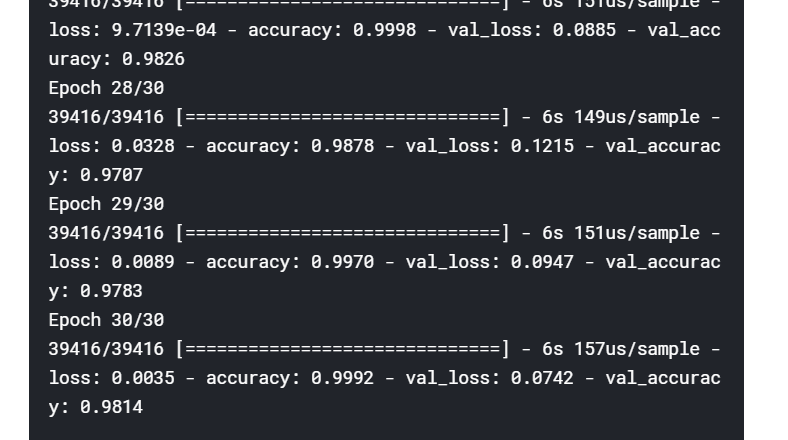（4）绘制训练和验证数据的准确性和损失曲线

``import pandas as pd``import matplotlib.pyplot as plt``pd.DataFrame(history.history).plot(figsize = (8,5))``plt.grid(True)``plt.gca().set_ylim(0,1)``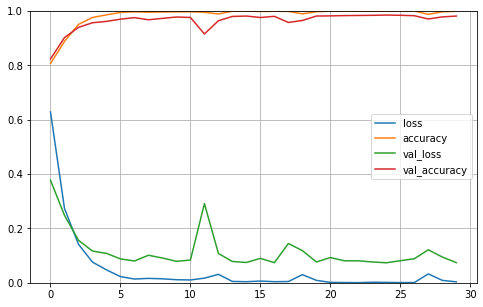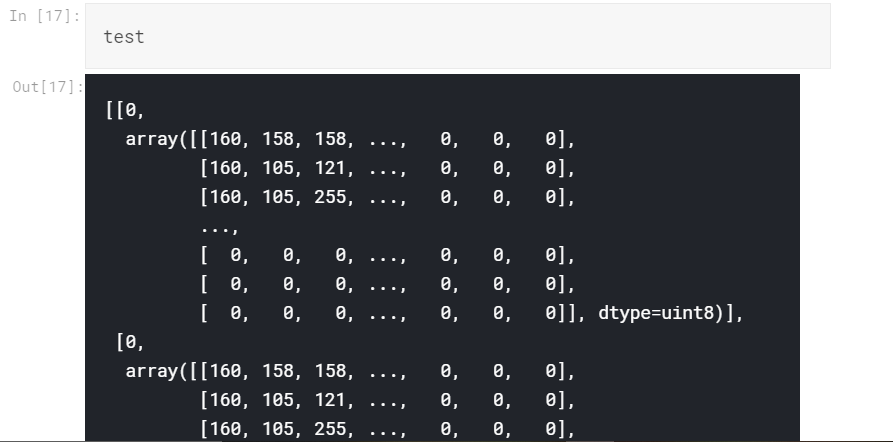````test_images, test_labels = [], []`
`for label, feature in test:``    test_images.append(feature)``    test_labels.append(label)``    ``test_images = np.array(test_images).reshape(-1, img_size, img_size, 1)``test_images = test_images / 255.0``del test``test_labels  = to_categorical(test_labels, num_classes = 2)````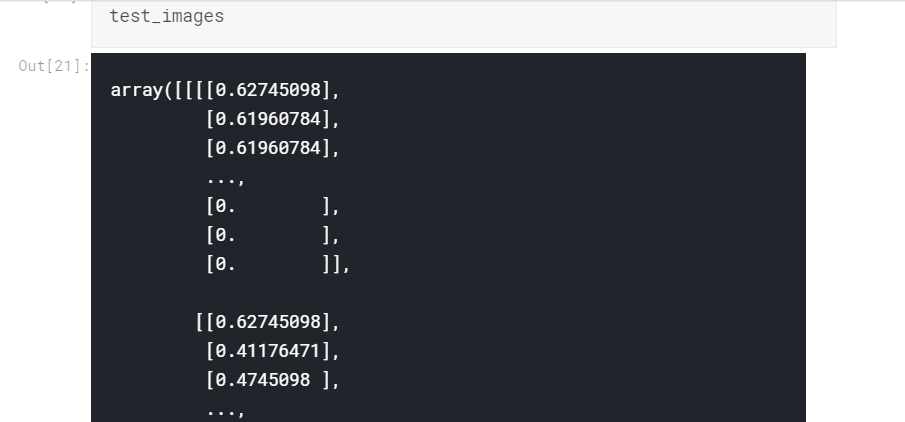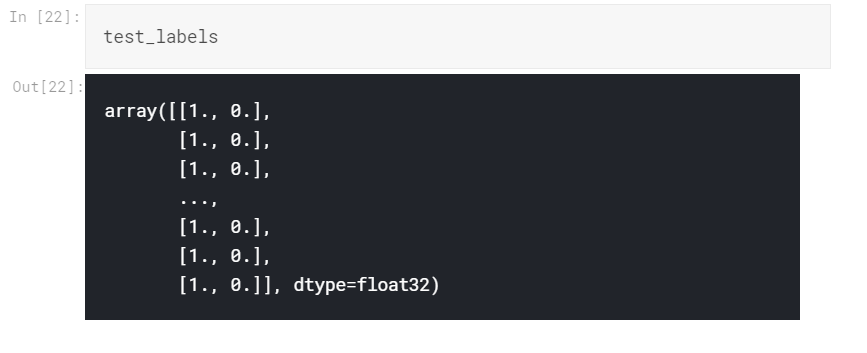``model.evaluate(test_images, test_labels)``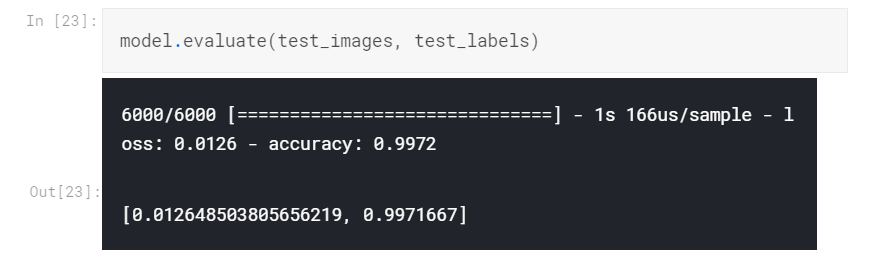https://www.kaggle.com/abolarinbukola/fingerprint-gender-classification-cnn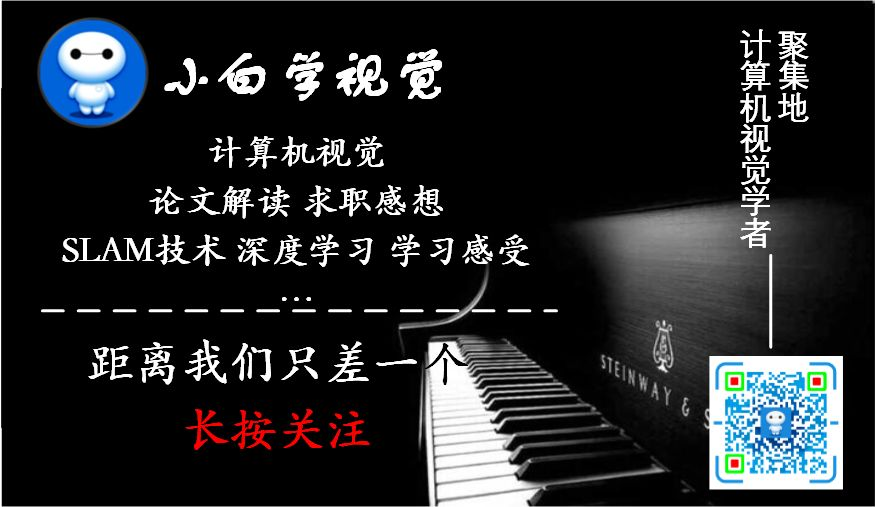2
19 收藏

### 作者的其它热门文章10/16 17:38

"我们只是不准备好迎接令人惊奇的事情。" 人类说话都这么机械了吗？
10/16 14:56

10/15 11:07

10/15 10:27

gif好评！
08/21 13:31

5 评论
19 收藏
2